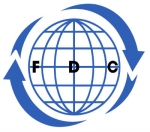Search all upcoming seminars, conferences, short management courses and training in Nigeria and around the WorldFinancial Modeling and Valuation Course

By: Foscore Development Center

Kenya

01 - 12 Apr, 2019  12 days

USD 2,000

The course extends the core theory introduced in Principles of Finance by emphasizing its practical application to strategic financial decisions and potential financial problems.

Who Should Attend?

• Financial Analysis
• Accountants
• Bookkeepers
• Investment officers
• Managing directors

Course Objective:

• Analyzing and understand an income statement
• Analyzing and understand a balance sheet
• Analyzing and understand a cash flow
• Understanding use of financial modeling in financial reporting
• Use of  Discounted Cash Flow (DCF) and Weighted Average Cost of Capital
• Use of excel in financial modelling

Course Content

Module 1: Valuation

• Introduction to valuation
• The importance of valuation
• Understanding enterprise value and equity value
• Comparable Company Analysis
• Selecting comparable companies
• Analyzing the valuation multiples
• Concluding and understanding value
• Precedent Transactions Analysis
• Selecting comparable transactions
• Discounted Cash Flow (DCF) analysis
• Understanding unlevered free cash flow
• Forecasting free cash flow
• Forecasting terminal value
• Present value and discounting
• Understanding stub periods
• Performing sensitivity analysis
• Weighted Average Cost of Capital (WACC)
• Using the CAPM to estimate the cost of equity
• Estimating the cost of debt
• Understanding and analyzing WACC

Module 2: Building an Integrated Cash Flow Model

• Introduction to financial modeling
• Understanding the links between the financial statements
• Understanding circularity
• Setting up and formatting the model
• Selecting model drivers and assumptions
• Modeling and projecting the financial statements
• Projecting the income statement
• Projecting the balance sheet
• Projecting the cash flow statement
• Creating the debt and interest schedule
• Revolver modeling
• Analyzing and concluding the model
• Analyzing the output
• Stress testing the model
• Fixing modeling errors
• Using the model to create a Discounted Cash Flow (DCF) Analysis

General Notes

• All our courses can be Tailor-made to participants needs
• The participant must be conversant with English
• Presentations are well guided, practical exercise, web based tutorials and group work. Our facilitators are expert with more than 10years of experience.
• Upon completion of training the participant will be issued with Foscore development center certificate (FDC-K)
 Foscore Development Center, Nairobi Kenya Apr 01 - 12 Apr, 2019

Class Session: 09:00:am - 16:30:pm

 USD 2,000.00
(Convert Currency)

Jackson Munene +254712260031

A discount of 10% to 50% for a group of more than two
Foscore Development Center